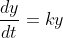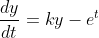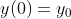# Question We know that with ample space and food resources, the rate of change of the population of a species may, at least for a while, be proportional to the population. Namely, Here the k > 0 term represents the proportionality constant. Now, assume that to make sure that our population doesn’t grow too much we harvest/remove some of the population at a certain time-dependent rate. Suppose that our new model for the population is, where g(t) = e^(t) is the rate at which we are harvesting. We see that this equation is neither autonomous nor separable. However, we can still solve it. One way of solving this problem is to exploit the properties of the equation. We can do it as follows: (a) Assume first that the solution is of the form y(t) = e^(t)*u(t). Then plug this into the equation and write a new differential equation in terms of the function u. (b) Solve the equation terms of u and find the answer to your original model by writing y(t) = e^(t)*u(t).We know that with ample space and food resources, the rate of change of the population of a species may,
at least for a while, be proportional to the population. Namely,Here the k > 0 term represents the proportionality constant. Now, assume that to make sure that our population doesn’t grow too much we harvest/remove some of the
population at a certain time-dependent rate. Suppose that our new model for the population is,where g(t) = e^(t) is the rate at which we are harvesting.

We see that this equation is neither autonomous nor separable. However, we can still solve it. One way of solving this problem is to exploit the properties of the equation. We can do it as follows:
(a) Assume first that the solution is of the form y(t) = e^(t)*u(t). Then plug this into the equation and write a new differential equation in terms of the function u.
(b) Solve the equation terms of u and find the answer to your original model by writing y(t) = e^(t)*u(t).

Transcribed Image Text: We know that with ample space and food resources, the rate of change of the population of a species may, at least for a while, be proportional to the population. Namely, Here the k > 0 term represents the proportionality constant. Now, assume that to make sure that our population doesn’t grow too much we harvest/remove some of the population at a certain time-dependent rate. Suppose that our new model for the population is, where g(t) = e^(t) is the rate at which we are harvesting. We see that this equation is neither autonomous nor separable. However, we can still solve it. One way of solving this problem is to exploit the properties of the equation. We can do it as follows: (a) Assume first that the solution is of the form y(t) = e^(t)*u(t). Then plug this into the equation and write a new differential equation in terms of the function u. (b) Solve the equation terms of u and find the answer to your original model by writing y(t) = e^(t)*u(t).
More
Transcribed Image Text: We know that with ample space and food resources, the rate of change of the population of a species may, at least for a while, be proportional to the population. Namely, Here the k > 0 term represents the proportionality constant. Now, assume that to make sure that our population doesn’t grow too much we harvest/remove some of the population at a certain time-dependent rate. Suppose that our new model for the population is, where g(t) = e^(t) is the rate at which we are harvesting. We see that this equation is neither autonomous nor separable. However, we can still solve it. One way of solving this problem is to exploit the properties of the equation. We can do it as follows: (a) Assume first that the solution is of the form y(t) = e^(t)*u(t). Then plug this into the equation and write a new differential equation in terms of the function u. (b) Solve the equation terms of u and find the answer to your original model by writing y(t) = e^(t)*u(t).&#12304;General guidance&#12305;The answer provided below has been developed in a clear step by step manner.Step1/2ANSWER:a-)To solve the given differential equation using the method mentioned in step 2, let's assume that the solution is of the form $$\mathrm{{y}{\left({t}\right)}={e}^{{{t}}}{u}{\left({t}\right)}}$$. Then, we have:$$\mathrm{{\frac{{{\left.{d}{y}\right.}}}{{{\left.{d}{t}\right.}}}}={e}^{{{t}}}{\frac{{{d}{u}}}{{{\left.{d}{t}\right.}}}}+{e}^{{{t}}}{u}}$$$$\mathrm{={k}{u}-{e}^{{{t}}}\quad\text{(from the given differential equation)}}$$Substituting $$\mathrm{{y}{\left({t}\right)}={e}^{{{t}}}{u}{\left({t}\right)}}$$ in the given differential equation, we get:$$\mathrm{{e}^{{{t}}}{\frac{{{d}{u}}}{{{\left.{d}{t}\right.}}}}+{e}^{{{t}}}{u}={k}{u}-{e}^{{{t}}}}$$$$\mathrm{{e}^{{{t}}}{\frac{{{d}{u}}}{{{\left.{d}{t}\right.}}}}={k}{u}-{2}{e}^{{{t}}}}$$$$\mathrm{{\frac{{{d}{u}}}{{{\left.{d}{t}\right.}}}}={k}{e}^{{-{t}}}{u}-{2}}$$Explanation\( \mathrm{\quad\text{(from the given differentia ... See the full answer